# Hasse principle

(diff) ← Older revision | Latest revision (diff) | Newer revision → (diff)
Letbe a class of algebraic varieties over a global field. The Hasse principle holds inif for anyinsuch that for all non-trivial absolute valuationsonthe set of-rational points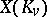ofis non-empty, the set of-rational points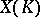is also not empty (where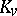is the completion ofrelative to). In particular, ifis the fieldof rational numbers, then if the set of real points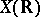and the set of-adic points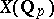, for all primes, are not empty, it follows that the set of rational points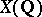is also not empty. The Hasse principle holds for quadrics , and so it is valid for algebraic curves of genus 0 (see ). For quadrics over a number field the Hasse principle was stated and proved by H. Hasse in . For cubic hypersurfaces the Hasse principle is not true, in general (see , ); a counterexample (over) is the projective curveor the projective surface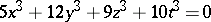.
Letbe an algebraic group overand let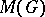be the class of algebraic varieties consisting of all principal homogeneous spaces over(see Galois cohomology; Weil–Châtelet group, and also , , ). One says that the Hasse principle holds forif it holds for. The Hasse principle holds for simply-connected and adjoint semi-simple algebraic groups over number fields (, ). Ifis an Abelian variety, then the Hasse principle holds forif and only if the Shafarevich–Tate group (cf. Galois cohomology) ofvanishes (see the examples in , ).